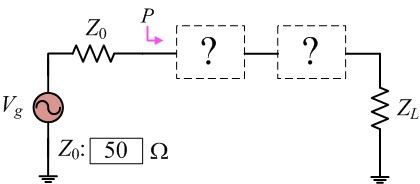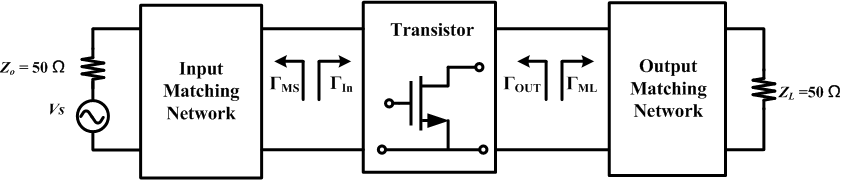Module 10.4 Matching Network

Mode  :     Input mode    Output modePlease input the Γ_(MS) Γ_(ML) : Γ_(MS) Γ_(ML)(mag∠phase) = ∠ Z_(MS) Z_(ML)   =    * ( +j Omega) Operating Frequency(F_{0})   =   GHz Operating Frequency(F_{0}) Range  =  0.1 GHz ~ 6GHz Shunt L   Shunt C   Series L   Series C   Series TL   Shunt Open Stub   Shunt Short Stub Shunt L   Shunt C   Series L   Series C   Series TL   Shunt Open Stub   Shunt Short Stub 匹配
*

## Introduction:

### Simultaneous conjugate match conditions:

The conditions required to obtain maximum transducer power gain are

Γ_(S) = Γ_(MS)= Γ_(IN)^**

Γ_(L) = Γ_(ML)= Γ_(OUT)^**

Γ_(S)^** = S_(11) + (S_(21) S_(12) Γ_(L) )/(1 - S_(22) Γ_(L) )

Γ_(L)^** = S_(22) + (S_(21) S_(12) Γ_(S) )/(1- S_(11) Γ_(S) )

Solving the above equations simultaneously, we obtain

Γ_(MS) = (B_(1) ± sqrt( B_(1)^2-4|C_(1)|^2 )) / (2C_(1))

Γ_(ML) = (B_(2) +- sqrt(B_(2)^2 - 4|C_(2)|^2 ))/ (2C_(2))

B_(1) = 1+|S_(11)|^2 -|S_(22)|^2 -|⧍|^2

B_(2) = 1+|S_(22)|^2 -|S_(11)|^2 -|⧍|^2

C_(1) = S_(11) -⧍S_(22)^**

C_(2) = S_(22) -⧍S_(11)^**

Γ_(MS) = |Γ_(MS)|e^(jθ_(Γ_(s))) = (Z_(MS)-Z_(0))/(Z_(MS)+Z_(0) )    Γ_(ML) = |Γ_(ML)|e^(jθ_(Γ_(L))) = (Z_(ML)-Z_(0))/(Z_(ML)+Z_(0) )

Γ_(MS) : Required input reflection coefficient for the simultaneous conjugate match.
Γ_(ML) : Required output reflection coefficient for the simultaneous conjugate match.
Z_(MS) : Required input Impedance for the simultaneous conjugate match
Z_(ML) : Required output Impedance for the simultaneous conjugate match### Simultaneous conjugate matching network design:

The impedance, Z_(MS), represented by the input reflection coefficient,
Γ_(MS), is the impedance seen into the input matching network.
Thus, the input matching network has to transform Z_(0) to the impedance Z_(MS).

The impedance, Z_(ML), represented by the output reflection coefficient,
Γ_(ML), is the impedance seen into the output matching network.
Thus, the output matching network has to transform Z_(0) to the impedance Z_(ML).